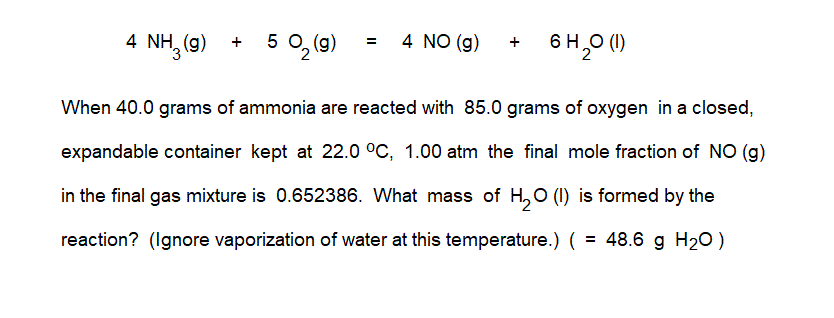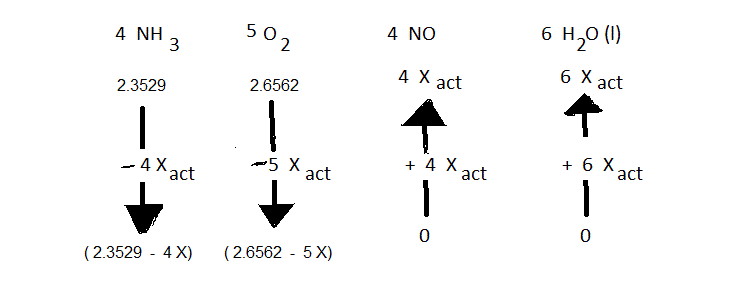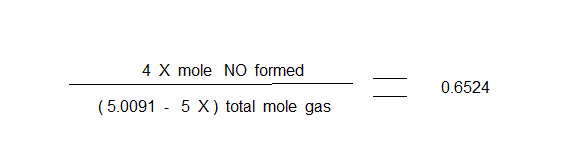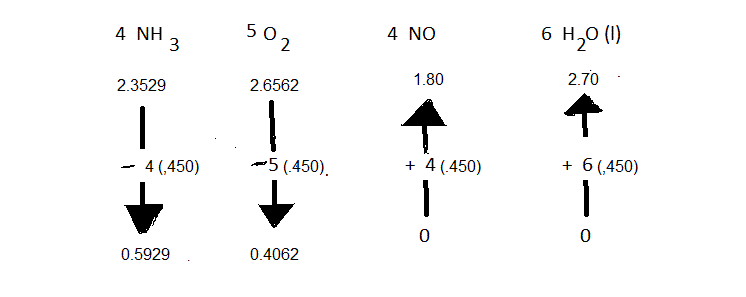(This page shows how the booger problem posted at medium.com can be worked out with the "arrow diagram" method. Additional worked out sample problems are shown at this website.)Conventional unit conversion calculations are done to convert the masses put in at the start of the reaction into moles. The student needs to be adept at using the given chemical formula of a compound and values from the Periodic Table to convert grams of compound into moles of compound. The preliminary set up of the a0rrow diagram for this problem looks like this:The given fact in the problem, in this case that the final mole fraction of the NO(g) in the final gas mixture is 0.6524, is expressed as an algebraic equation that can be solved for the value of " X " in the arrow diagram:Solving this equation givesX = 0.4500

The arrow diagram with the value of X being used at all appropriate places is thenTo answer the question, then, it is only necessary to change moles of water formed (2.70 mole H2O) into grams.